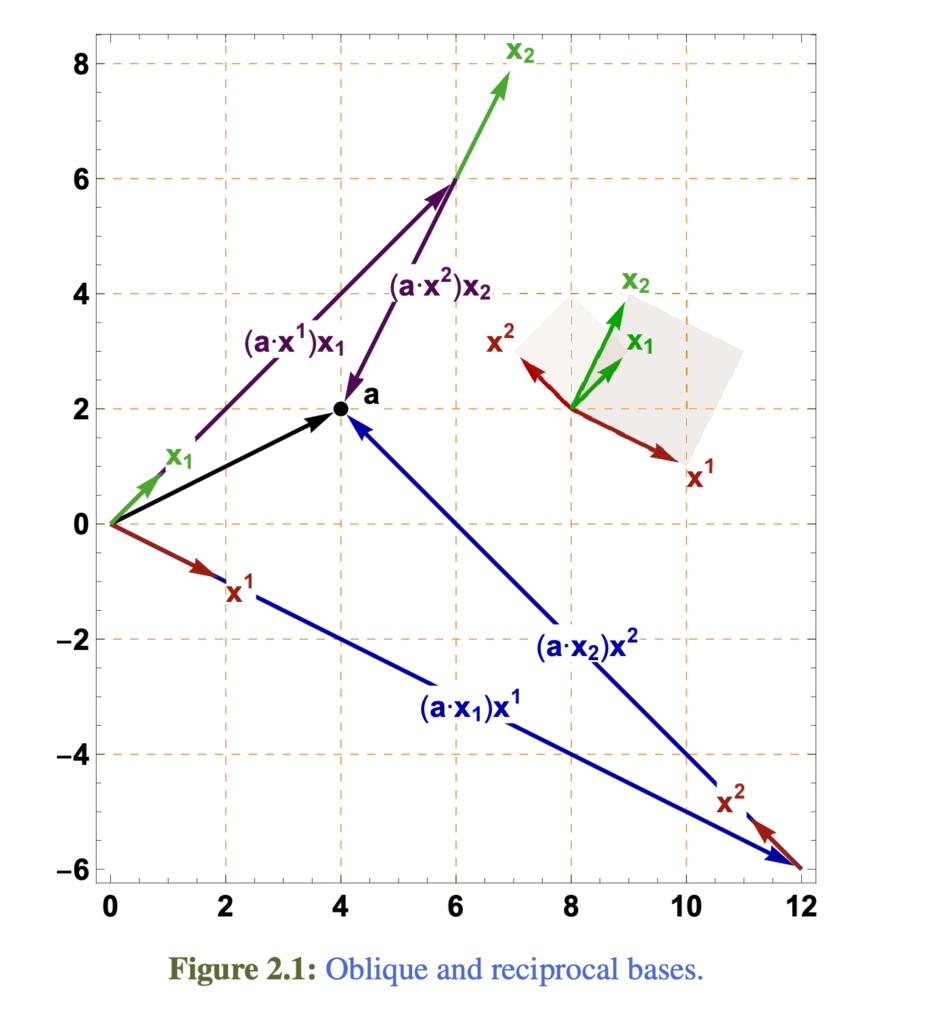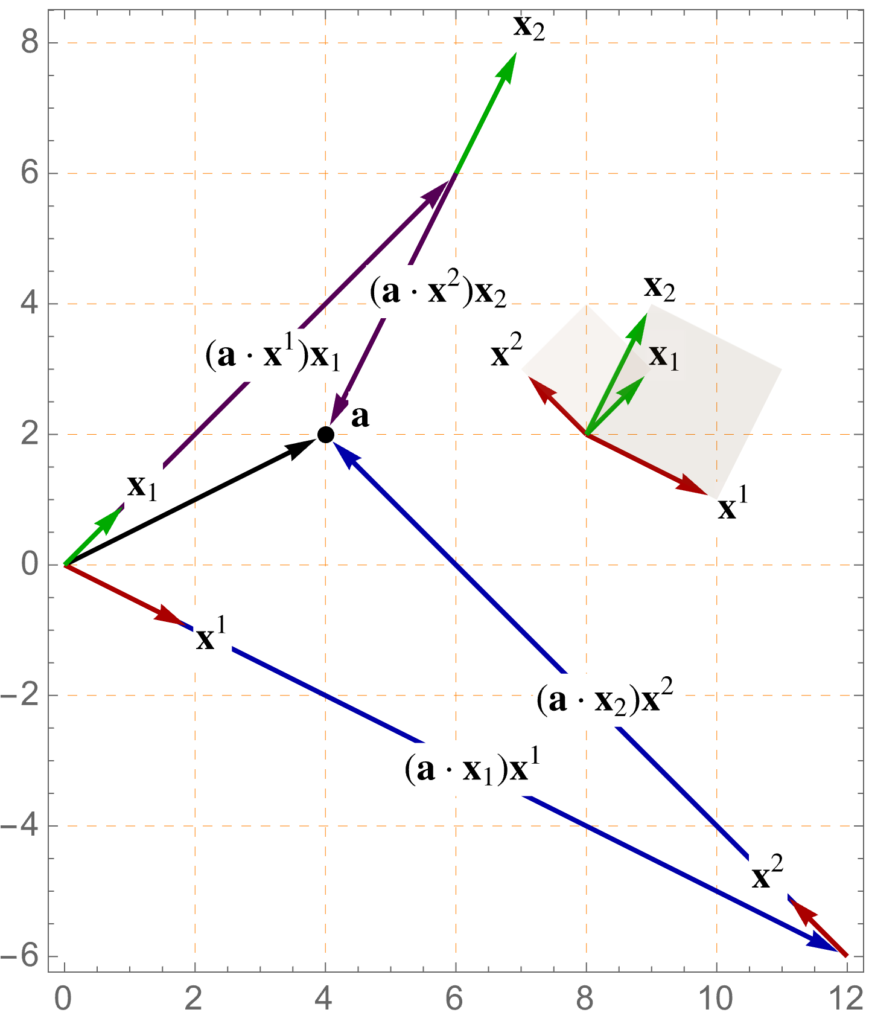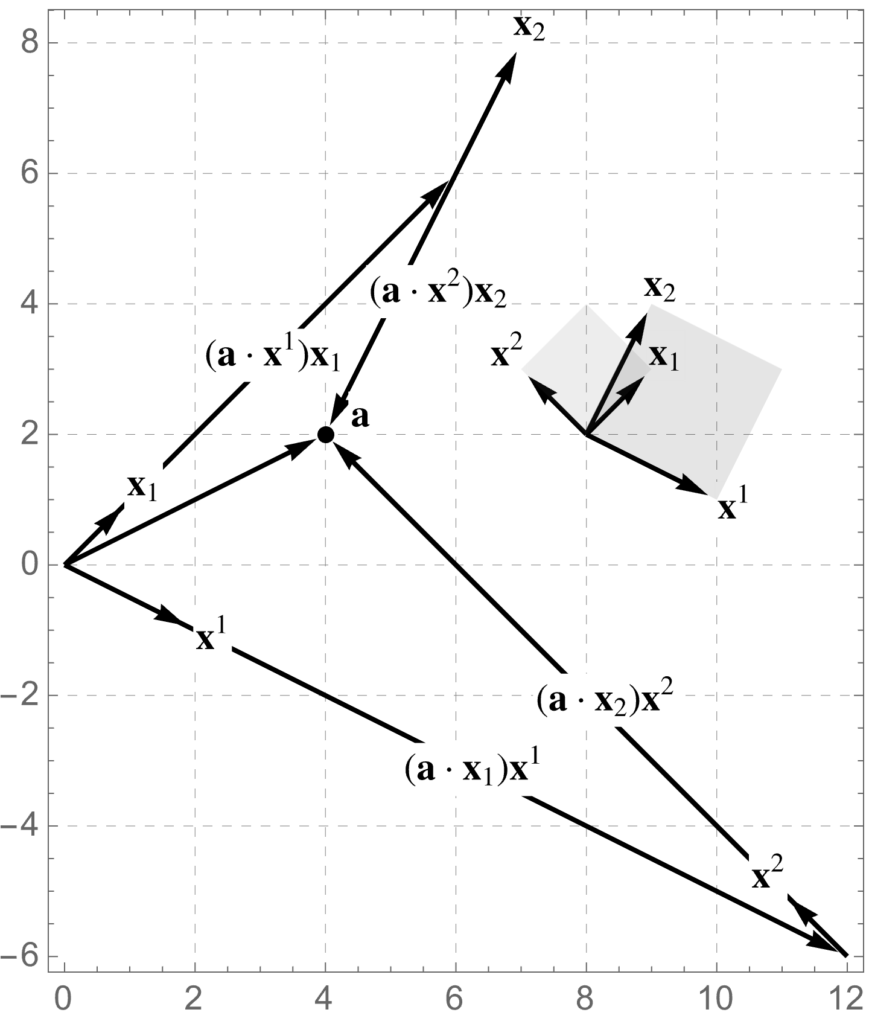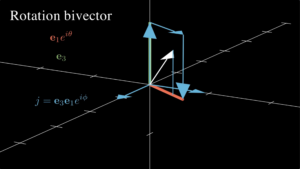## Updated figures in ‘Geometric Algebra for Electrical Engineers’

September 2, 2023 Geometric Algebra for Electrical Engineers , , ,

New version of the book is now published (online PDF and leanpub versions updated, with amazon updates in the approval pipeline)

• V0.1.19-2 (Sep 2, 2023)
• Reworked many of the Mathematica generated figures.  Now using the MaTeX[] extension to do the figure labelling (that was only done in a couple figures before this), as it looks much better, and is consistent with the fonts in the text.

Each of these are individually very small changes, barely noticeable, but I think it makes a nice difference to overall quality.

In many cases, I’ve generated new separate figures for the amazon paper editions of the book, using straight black instead of colors, so they don’t look as washed out, after conversion to black and white.

Here’s an example where just the captioning was changed:

The font is now whatever LaTeX uses for \\mathbf{n}, so it matches the text.

I think that the new Mathematica version (13.2) that I am using, also happens to render this 3D figure a bit nicer.

Here’s a comparison of one of the figures that now has a black and white specialization (old, new-color, new-bw):In this particular case, I chose not to color the labels like I did previously, but I have retained that label color matching in some places.

Like I said, it’s a small difference, but the latex labelling just look better, period.  Notice that the numeric values at the tick marks on the border of the figure are not using a matching font (those are directly generated by Mathematica).  I’ll have to figure out how to make those use MaTeX too, and audit all the figures for that, but that’s a game for another day.

## Video: Spherical basis vectors in geometric algebraI’ve made a new manim-based video with a geometric algebra application.

In the video, the geometric algebra form for the spherical unit vectors are derived, then unpacked to find the conventional vector algebra form. We will then use our new tools to find the expression for the kinetic energy of a particle in spherical coordinates.

Prerequisites: calculus (derivatives and chain rule), complex numbers (exponential polar form), and geometric algebra basics (single sided rotations, vector multiplication, vector commutation sign changes, …)

You can find the video on Google’s censorship-tube, or on odysee.

## New revision of my book: Geometric Algebra for Electrical Engineers

May 16, 2022 Geometric Algebra for Electrical Engineers

A new version of my book is now available in free pdf form, in paperback and hardcover on amazon, on leanpub, and for the brave or crazy — as latex source files and a makefile.  All of these can be found here:

Geometric Algebra for Electrical Engineers.

Changes in this V0.1.16-13 (May 15, 2022) version, include:

• Fix equations 2.81 2.82 — error found by Christopher
• test drove build instructions (slight fix required.)
• restore latexsym \Box as dAlembertian
• gutter fixes.
• perl -p -i -e ‘s/ *\$//’ cat spellcheckem.txt
• (massive change with no visible effects if done right): purge most dmath usage. MacTex upgrade has made these seemingly malfunction, and lines are getting split in all sorts of weird places.

## [Part 1. Arrow representation of vectors] An introduction to geometric algebra.

August 2, 2020 Geometric Algebra for Electrical Engineers

This is a continuation of:

# Vectors.

Cast yourself back in time, all the way to high school, where the first definition of vector that you would have encountered was probably very similar to the one made famous by the not very villainous Vector in Despicable Me .  His definition was not complete, but it is a good starting point:

### Definition: Vector. A vector is a quantity represented by an arrow with both direction and magnitude.

All the operations that make vectors useful are missing from this definition, such as

• a comparison operator,
• a rescaling operation (i.e. a scalar multiplication operation that changes the length),
• an operator that provides the length of a vector,
• multiplication or multiplication like operations.

The concept of vector, once supplemented with the operations above, will be useful since it models many directed physical quantities that we experience daily.  These include velocity, acceleration, forces, and electric and magnetic fields.

## Vector comparison.

In fig. 1.1 (a), we have three vectors, labelled $$\Ba, \Bb, \Bc$$, all with different directions and magnitudes, and in fig. 1.1 (b), those vectors have each been translated (moved without rotation or change of length) slightly. Two vectors are considered equal if they have the same direction and magnitude. That is, two vectors are equal if one is the image of the other after translation. In these figures $$\Ba \ne \Bb, \Bb \ne \Bc, \Bc \ne \Ba$$, whereas any same colored vectors are equal.

## Vector (scalar) multiplication.

We can multiply vectors by scalars by changing their lengths appropriately.

In this context a scalar is a real number (this is purposefully vague, as it will be useful to allow scalars to be complex valued later.)

Using the example vectors, some rescaled vectors include $$2 \Ba, (-1) \Bb, \pi \Bc$$, as illustrated in fig. 1.2.

Scalar multiplication implicitly provides an algorithm for addition of vectors that have the same direction, as $$s \Bx + t \Bx = (s+t) \Bx$$ for any scalars $$s, t$$. This is illustrated in fig. 1.3 where $$2 \Ba = \Ba + \Ba$$ is formed in two equivalent forms. We see that the addition of two vectors that have the same direction requires lining up those vectors head to tail. The sum of two such vectors is the vector that can be formed from the first tail to the final head.

It turns out that this arrow daisy chaining procedure is an appropriate way of defining addition for any vectors.

### Definition: Vector addition. The sum of two vectors can be found by connecting those two vectors head to tail in either order. The sum of the two vectors is the vector that can be formed by drawing an arrow from the initial tail to the final head. This can be generalized by chaining any number of vectors and joining the initial tail to the final head.

This addition procedure is illustrated in fig. 1.4, where $$\Bs = \Ba + \Bb + \Bc$$ has been formed.

## Vector subtraction.

Since we can scale a vector by $$-1$$ and we can add vectors, it is clear how to define vector subtraction

### Definition: Vector subtraction. The difference of vectors $$\Ba, \Bb$$ is \begin{equation*} \Ba – \Bb \equiv \Ba + ((-1)\Bb). \end{equation*}

Graphically, subtracting a vector from another requires flipping the direction of the vector to be subtracted (scaling by $$-1$$), , and then adding both head to tail. This is illustrated in fig. 1.5.

## Length and what’s to come.

It is easy to compute the length of a vector that has an arrow representation.
One simply lines a ruler of appropriate units along the vector and measures.

We actually want an algebraic way of computing length, but there is some baggage required, including

• Coordinates.
• Bases (plural of basis).
• Linear dependence and independence.
• Dot product.
• Metric.

The next part of this series will cover these topics. Our end goal is geometric algebra, which allows for many coordinate free operations, but we still have to use coordinates, both to read the literature, and in practice. Coordinates and non-orthonormal bases are also a good way to introduce non-Euclidean metrics.

# References

 Vector; supervillain extraordinaire (Despicable Me). A quantity represented by an arrow with direction and magnitude. Youtube. URL https://www.youtube.com/watch?v=bOIe0DIMbI8. [Online; accessed 11-July-2020].

## What’s in the pipe.

It’s been a while since I did any math or physics writing. This is the first post in a series where I plan to work my way systematically from an introduction of vectors, to the axioms of geometric algebra.  I plan to start with an introduction of vectors as directed “arrows”, building on that to discuss coordinates, tuples, and column matrix representations, and representation independent ideas. With those basics established, I’ll remind the reader about how generalized vector and dot product spaces are defined and give some examples. Finally, with the foundation of vectors and vector spaces in place, I’ll introduce the concept of a multivector space, and the geometric product, and start unpacking the implications of the axioms that follow naturally from this train of thought.

The applications that I plan to include in this series will be restricted to Euclidean spaces (i.e. where length is given by the Pythagorean law), primarily those of 2 and 3 dimensions.  However, it will be good to also lay the foundations for the non-Euclidean spaces that we encounter in relativistic electromagnetism (there is actually no other kind), and in computer graphics applications of geometric algebra, especially since we can do so nearly for free.  I plan to try to introduce the requisite ideas (i.e. the metric, which allows for a generalized dot product) by discussing Euclidean non-orthonormal bases.  Such bases have applications in condensed matter physics where there are useful for modelling crystal and lattice structure, and provide a hands conceptual bridge to a set of ideas that might otherwise seem abstract and without “real world” application.

## Motivation.

Many introductions to geometric algebra start by first introducing the dot product, then bivectors and the wedge product, and eventually define the product of two vectors as the synthetic sum of the dot and wedge
\begin{equation}\label{eqn:multivector:20}
\Bx \By = \Bx \cdot \By + \Bx \wedge \By.
\end{equation}
It takes a fair amount of work to do this well. In the seminal work  a few pages are taken for each of the dot and wedge products, showing the similarities and building up ideas, before introducing the geometric product in this fashion. In  the authors take a phenomenal five chapters to build up the context required to introduce the geometric product.  I am not disparaging the authors for taking that long to build up the ideas, as their introduction of the subject is exceedingly clear and thorough, and they do a lot more than the minumum required to define the geometric product.

The strategy to introduce the geometric product as a sum of dot and wedge can result in considerable confusion, especially since the wedge product is often defined in terms of the geometric product
\begin{equation}\label{eqn:multivector:40}
\Bx \wedge \By =
\inv{2} \lr{
\Bx \By – \By \Bx
}.
\end{equation}
The whole subject can appear like a chicken and egg problem. I personally found the subject very confusing initially, and had considerable difficulty understanding which of the many identities of geometric algebra were the most fundamental. For this reason, I found the axiomatic approach of  very refreshing. The cavaet with that work is that is is exceptionally terse, as they jammed a reformulation of most of physics using geometric algebra into that single book, and it would have been thousands of pages had they tried to make it readable by mere mortals.

When I wrote my own book on geometric algebra, I had the intuition that the way to introduce the subject ought to be like the vector space in abstract linear algebra. The construct of a vector space is a curious and indirect way to define a vector. Vectors are not defined as entities, but simply as members of a vector space, a space that is required to have a set of properties. I thought that the same approach would probably work with multivectors, which could be defined as members of a multivector space, a mathematical construction with a set of properties.

I did try this approach, but was not fully satisfied with what I wrote. I think that dissatisfaction was because I tried to define the multivector first. To define the multivector, I first introduced a whole set of prerequisite ideas (bivector, trivector, blade, k-vector, vector product, …), but that was also problematic, since the vector multiplication idea required for those concepts wasn’t fully defined until the multivector space itself was defined.

My approach shows some mathematical cowardness. Had I taken the approach of the vector space fully to heart, the multivector could have been defined as a member of a multivector space, and all the other ideas follow from that. In this multi-part series, I’m going to play with this approach anew, and see how it works out.  If it does work, I’ll see if I can incorporate this approach into a new version of my book.

## Review and background.

In this series, I’m going to assume a reader interested in geometric algebra, is probably also familiar with a wide variety of concepts, including but not limited to

• vectors,
• coordinates,
• matrices,
• basis,
• change of basis,
• dot product,
• real and complex numbers,
• rotations and translations,
• vector spaces, and
• linear transformations.

Despite those assumptions, as mentioned above, I’m going to attempt to build up the basics of vector representation and vector spaces in a systematic fashion, starting from a very elementary level.

My reasons for doing so are mainly to explore the logical sequencing of the ideas required.  I’ve always found well crafted pedagogical sequences rewarding, and will hopefully construct one here that is appreciated by anybody who chooses to follow along.

## Next time.

As preparation for the next article in this series, the reader is asked to watch a short lesson from Vector, not so supervillain extraordinaire (Despicable Me).

## References

 C. Doran and A.N. Lasenby. Geometric algebra for physicists. Cambridge University Press New York, Cambridge, UK, 1st edition, 2003.

 L. Dorst, D. Fontijne, and S. Mann. Geometric Algebra for Computer Science. Morgan Kaufmann, San Francisco, 2007.

 D. Hestenes. New Foundations for Classical Mechanics. Kluwer Academic Publishers, 1999.